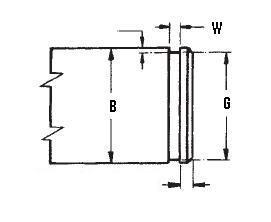### XAN-098

Display Units: inches |  metric
Request Quick Quote for this part.
 Ring Specs (D) Free Diameter: 0.925 +0.000 / -0.031 in. (t) Thickness: 0.042 +0.002 / -0.002 in. (b) Radial Wall: 0.078 +0.003 / -0.003 in. Groove Specs: (B) Application Diameter: 0.984 in. (G) Groove Diameter: 0.940 +0.003 / -0.003 in. (W) Groove Width: 0.046 +0.003 / -0.000 in. Groove Depth: 0.022 in. Other Specs Approximate Weight per 1000: (FG Max) Free Gap Max.: 0.250 in. (FG min) Free Gap Min.: 0.093 in. (Pg) Theoretical Thrust Load Capacity - Groove Yield:Notes: Yield Strength of Groove Material (Ys): 45,000 psi. Calculated using a safety factor (K) of 4Equation:Pg = [ B * d * Ys * pi ] / K    = [ (0.984 in.) * (0.022 in.) * (45,000 psi) * 3.14 ] / 4 764 lbs. (Pr) Theoretical Thrust Load Capacity - Ring Shear:Notes: Ring Material: Carbon Spring Steel (SAE 1060-1095). Shear Strength of Ring Material (Ss): 138,000 psi. Calculated using a safety factor (K) of 3Equation:Pr = [ B * t * Ss * pi ] / K    = [ (0.984 in.) * (0.042 in.) * (138,000 psi) * 3.14 ] / 3 5,969 lbs. Industry Equivalent Part Number(s):XAN-098, XAN-98